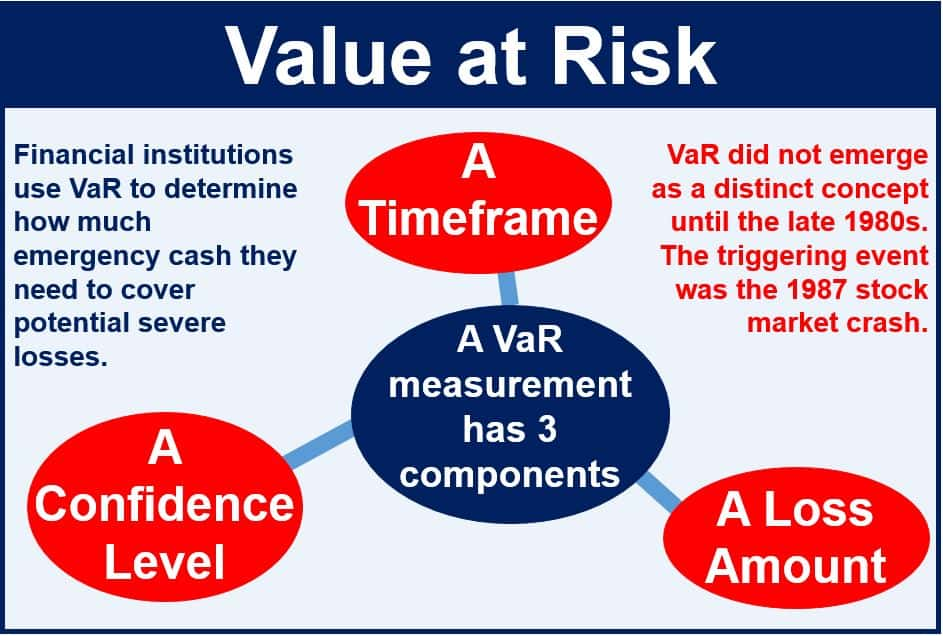What is value at risk (VaR)? Definition and meaning

Value at risk (VaR) is used to measure the risk of loss on a portfolio of financial assets, or an investment, over a specific period. Financial institutions use VaR to determine how much emergency cash they need to put aside to cover potential losses.

It has sometimes been referred to as the “new science of risk management”. VaR is mainly used in risk management, financial control, financial reporting and computing regulatory capital.

VaR helps investors determine what could happen in a worst-case scenario – it tells them how much they could potentially lose in a really bad month, week or even day.VaR was developed as a systematic way to segregate extreme events.

It is the probability that a portfolio will experience a mark-to-market loss that exceeds that of a specific predetermined threshold value.

Essentially this means that value at risk is measured in three variables:

1. The amount of potential loss,
2. The probability of that loss, and
3. The timeframe.

The most common parameters for VaR are 1%, 5%, and 10% probabilities and time periods of one day, two weeks, or a month. However, there are a variety of different combinations that investors use.

Example of value at risk (VaR)

If a portfolio has a VaR of 10% on a certain day of \$10 million USD, then this portfolio has a 0.10 probability that the portfolio will drop in value by \$10 million. A loss of more than the VaR threshold is considered to be a “VaR break”.

Video – Value at Risk (VaR)